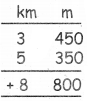Students can download 5th Maths Term 1 Chapter 4 Measurements Ex 4 Questions and Answers, Notes, Samacheer Kalvi 5th Maths Guide Pdf helps you to revise the complete Tamilnadu State Board New Syllabus, helps students complete homework assignments and to score high marks in board exams.

## Tamilnadu Samacheer Kalvi 5th Maths Solutions Term 1 Chapter 4 Measurements Ex 4

Question 1.
Fill in the blanks.

a. 7m 5cm = ______ cm
7m 5cm = 7 × 100 + 5cm: 700 + 5 = 705 cm

b. 505 mm = ______ cm ______ mm
505 mm = 505 + 100 = 50 cm 5 mmc. 326m = ______ cm
326 m = 326 × 100 = 32600 cm

d. 5km 30m = _____ m
5 km 30 m : 5 × 1000 + 30 = 5000 + 30 = 5030 m

e. 650cm: ______ m ______ cm
650 cm = 650 ÷ 100 = 6 m 50 cm

Question 2.
True or false.

a) 600 m is 6mm.
False (∵6 cm)

b) 7000 m is 7 km.
Truec) 400 cm is 4 km.
False (∵4m)

d) 770 mm is 77 cm.
True

e) 9000m is 90 mm.
False (∵ 9000 × 1000 = 9000000 mm)

Question 3.
Find the sum of the following

a. 17 m 45 cm + 52 m 30 cm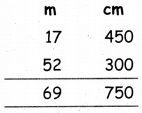= 69 m 750 cmb. 75 km 400 m + 37 km 300 m + 52 km 750 m
400 + 300 + 750 = 1450 m
1450 m = 1 km 450 m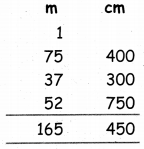= 165 km 450 m

c. 4 cm 8 mm + 5 cm 9 mm
8mm + 9mm = 17 mm = 1 cm 7 mm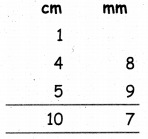= 1 cm 7mmQuestion 4.
Subtract the following

a. 15 km 450 m – 13 km 200 m.
Difference = 15 km 450 m – 13 km 200 m
= 2 km 250 m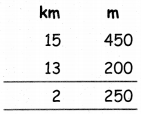b. 750 m 840 mm – 370m 480 mm.
Difference = 750 m 840 mm – 370m 480 mm
= 380 m 360 mm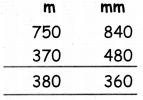c. 5 km 400 m – 3 km 350 m
Difference = 5 km 400 m – 3 km 350 m
= 2 km 50 mm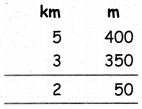Question 5.
Multiply the following.

a. 350 m 45c m × 7
45 × 7 = 315 m
= 3 m 15 m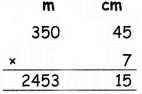350 m 45 cm × 7 = 2453 km 45 cm

b. 25 km 300 m × 6
300 × 6 = 1800 m
= 1 km 800 m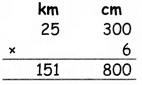25 km 300 m × 6 = 151 km 800 mm

c. 37 m 350 mm × 8
350 × 8 = 2800 mm
= 2 m 800 mm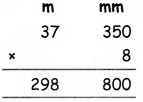37 m 350 mm × 8 = 298 m 800 mmQuestion 6.
Divide the following

a. 950 km 800 m ÷ 5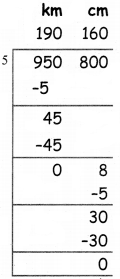950 km 800 ÷ 5 = 190 km 160 m

b. 49 m 770 mm ÷ 7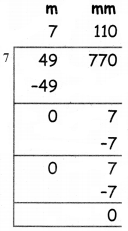49 m 770 mm ÷ 7 = 7 m 110 mm

c. 172 m 48 cm ÷ 4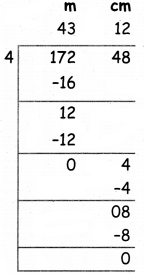172 m 48 cm ÷ 4 = 43 m 12 cmLife Related Problems

Question 7.

a. Saravanan had chosen to drive his vehicle from puducherry to Chennai for a distance of 165 Km. While starting his vehicle, the odometre showed 000157 Km, Find the reading of the odometre, when he reach Chennai?
Distance form Pudicherry to Chennai = 165 km
Odometer showed = 000157 km
Reading of odometer after reached Chennai = 165 km + 000157 km
= 000322 km.

b. Karthik Raja decided to travel from A. He moves lKm in east to reach B. Then he goes 2 Km towards north and reaches C. Then he goes 1 Km towards west and reaches D. If he goes 2 Km towards South, Where would he reach? Draw apt Diagram and Justify. Also find out the total distance travelled by him.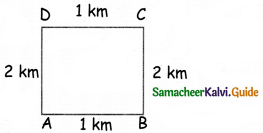He would reach = A
Total Distance =1 + 2 + 1 +2 = 6 kmc. Sangeetha has just finished building a new house with garden area. She measured the garden area and found it to be 6m x 6m. Suppose she has to put a pole every lm, how many poles are required? Each pole is of height 1.5m from the ground, What should be the total length of the fencing material to fence the garden?
Side of the Garden = 6m
No. of poles required = 6 + 6 +6+ 6= 24
The total length of the fencing material to fence the garden
= 24 × 1.5
= 36 m

d. One student needs lm 25 cm cloth to stich a shirt. What is the total length of clothes need to stitch a class of 22 students?
Cloth is needed for one student = 1 m 25 cm
Total cloth is needed for 22 students
= 1 m 25 cm × 22
= 27 m 50 cm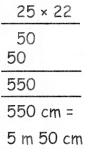e. The distance from village A to village B is 3 km 450 m. The distance from village B to village C is 5 km 350 m. What will be the total length of the road, if the road is laid from village A to village C?